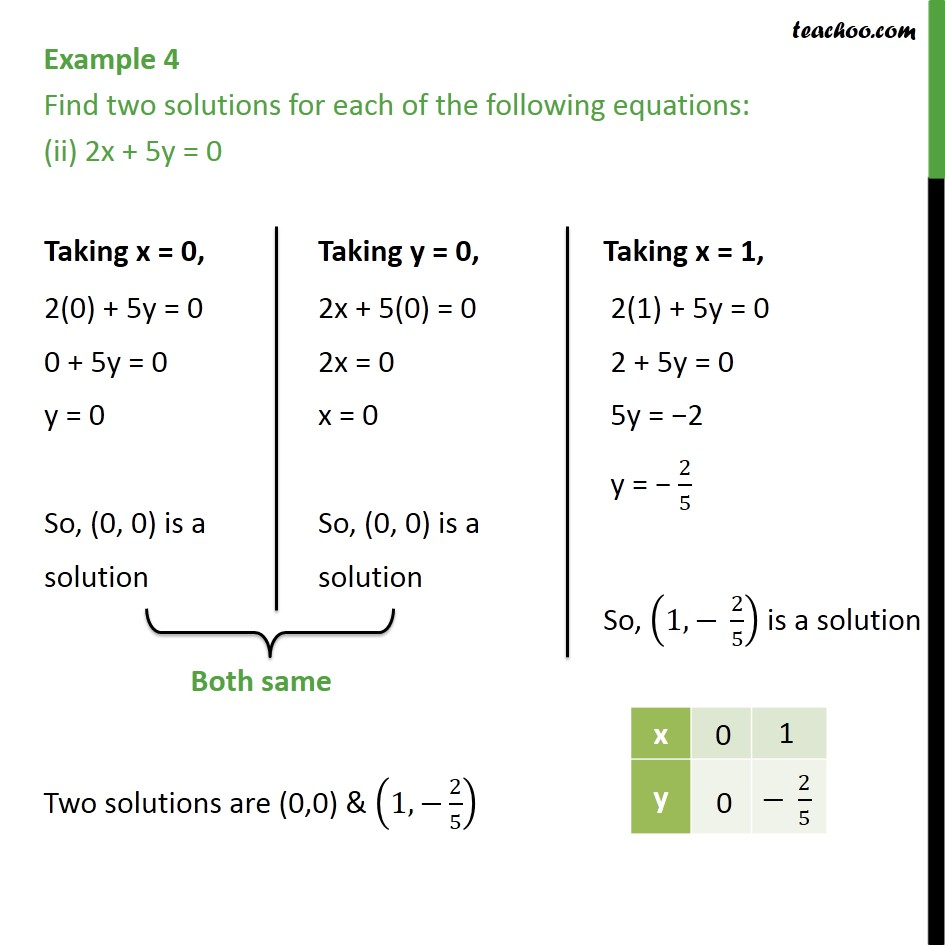Examples

Chapter 4 Class 9 Linear Equations in Two Variables
Serial order wiseLearn in your speed, with individual attention - Teachoo Maths 1-on-1 Class

### Transcript

Example 4 Find two solutions for each of the following equations: (ii) 2x + 5y = 0 Two solutions are (0,0) & (1, 2/5)